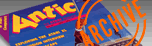`ANTIC VOL. 8, NO. 3 / JULY 1989`

# Tech Tips

GOTO LIST

BY GREGG HESLING

Don't type the TYPO II Codes!

```
DF 10 REM GOTOLIST
AA 20 REM BY GREGG HESLING
QH 30 REM (C)1988. ANTIC PUBLISHING
GF 31990 CLR :A=INT((FRE(0)-1000)/19)*19:
DIM A\$(A),B\$(7):A\$=" ":A\$(A)=A\$:A\$(2)=
A\$:A=1:B=PEEK(136)+PEEK(137)*256
BP 31991 LINE=PEEK(B)+PEEK(B+1)*256:C=1:I
F LINE=31998 THEN ? A\$:END
LQ 31992 IF C=PEEK(B+2) THEN B=B+PEEK(B+2
):GOTO 31991
MK 31993 PK0=PEEK(B+C):PK1=PEEK(B+C+1):IF
(PK1<>14 AND PK1<128) OR (PK0=27 AND
(PK1>=128) THEN 31999
JV 31994 RESTORE :FOR E=1 TO 8:READ D,B\$:
IF PK0<>D THEN NEXT E:GOTO 31999
HS 31995 IF PK0=30 THEN D=PEEK(B+C+2):D=(
D=23 OR D=24)*2:C=C+D:IF D=0 THEN 3199
9
KS 31996 PK1=PEEK(B+C+1):PK2=PEEK(B+C+2)-
61:IF PK1>=128 OR PK2<0 THEN A\$(A)="VA
R ":GOTO 31998
CN 31997 G=0:FOR D=3 TO PK2:E=PEEK(B+C+D)
:F=INT(E/16):G=G+((E+F*10-F*16)*INT(10
0^(PK2-D)+0.5)):NEXT D:A\$(A)=STR\$(G)
MC 31998 A\$(A+6)=B\$:A\$(A+14)=STR\$(LINE):A
=A+19:C=C+2+6*(PK1<128):IF PEEK(B+C)=1
8 THEN 31996
VG 31999 C=C+l:GOTO 31992:DATA 4,LIST,10,
GOTO,11,GO TO,12,GOSUB,13,TRAP,27,THEN
,30,ON,35,RESTORE
```

Recently I purchased an unprotected BASIC program and found it was mostly "spaghetti code" - a mindless collection of GOTO, GOSUB, and TRAP statements! I immediately decided to re-write it, but it was too long and too complicated.

I was deathly afraid to change any lines, fearing another line would try to call it! Because it was a BBS program, I couldn't have some user get a READY prompt while I wasn't home!

In my typical fashion of trying to write programs which I understand nothing about, I seem to have succeeded with GOTOLIST.

GOTOLIST will search any BASIC program and find all line numbers referenced by other program lines. For example, GOTOLIST would save line 10 if it is:

10 GOTO 100

Although GOTOLIST is small, it's not stupid. If it encounters the following line:

10 GRAPHICS 0:? "HELLO.";:INPUT A\$:IF AS= "NO" THEN ? "OK":GOTO 100

all it saves is: 10 GOTO 100.

Type in the listing below, GOTOLIST.LST, check it with TYPO II, and list a copy to disk with the command:

LIST "D:GOTOLIST.LST", 31990,31999

Now type NEW and LOAD a copy of that favorite program you've been dying to modify but are afraid of destroying. (Never modify your original copy-you're just asking for trouble!)

First, make sure your program doesn't use any line numbers above 31990. Then ENTER "D:GOTOLIST.LST" [RETURN] and type GOTO 31990. In a short while (It takes about a minute to check your program, so bring a good book if your program is long) GOTOLIST will display its list of lines which call other program lines.

If you want to see the list again, type PRINT A\$, or LPRINT A\$ for a printout. For best results, put your printer in 76-column mode by typing:

LPRINT CHR\$(27);CHR\$(81);CHR\$(76)